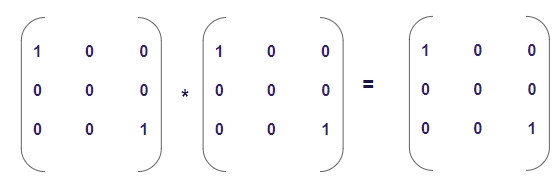# Program to check idempotent matrix in C++

C++Server Side ProgrammingProgramming

#### C in Depth: The Complete C Programming Guide for Beginners

45 Lectures 4.5 hours

#### Practical C++: Learn C++ Basics Step by Step

Most Popular

50 Lectures 4.5 hours

#### Master C and Embedded C Programming- Learn as you go

66 Lectures 5.5 hours

Given a matrix M[r][c], ‘r’ denotes number of rows and ‘c’ denotes number of columns such that r = c forming a square matrix. We have to check whether the given square matrix is an Idempotent matrix or not.

Idempotent Matrix

A matrix ‘M’ is called Idempotent matrix if and only the matrix ‘M’ multiplied by itself returns the same matrix ‘M’ i.e. M * M = M.

Like in the given example below −We can say that the above matrix is multiplied by itself and returns the same matrix; hence the matrix is Idempotent matrix

## Example

Input: m = { {2, -2, -4},
{-1, 3, 4},
{1, -2, -3}}
Output: Idempotent
Input: m == { {3, 0, 0},
{0, 2, 0},
{0, 0, 3} }
Output: Not Idempotent

## Algorithm

Start
Step 1 -> define macro as #define size 3
Step 2 -> declare function for matrix multiplication
void multiply(int arr[][size], int res[][size])
Loop For int i = 0 and i < size and i++
Loop For int j = 0 and j < size and j++
Set res[i][j] = 0
Loop for int k = 0 and k < size and k++
Set res[i][j] += arr[i][k] * arr[k][j]
End
End
End
Step 3 -> declare function to check idempotent or not
bool check(int arr[][size])
declare int res[size][size]
call multiply(arr, res)
Loop For int i = 0 and i < size and i++
Loop For int j = 0 and j < size and j++
IF (arr[i][j] != res[i][j])
return false
End
End
End
return true
step 4 -> In main()
declare int arr[size][size] = {{1, -1, -1},
{-1, 1, 1},
{1, -1, -1}}
IF (check(arr))
Print its an idempotent matrix
Else
Print its not an idempotent matrix
Stop

## Example

#include<bits/stdc++.h>
#define size 3
using namespace std;
//matrix multiplication.
void multiply(int arr[][size], int res[][size]){
for (int i = 0; i < size; i++){
for (int j = 0; j < size; j++){
res[i][j] = 0;
for (int k = 0; k < size; k++)
res[i][j] += arr[i][k] * arr[k][j];
}
}
}
//check idempotent or not
bool check(int arr[][size]){
int res[size][size];
multiply(arr, res);
for (int i = 0; i < size; i++)
for (int j = 0; j < size; j++)
if (arr[i][j] != res[i][j])
return false;
return true;
}
int main(){
int arr[size][size] = {{1, -1, -1},
{-1, 1, 1},
{1, -1, -1}};
if (check(arr))
cout << "its an idempotent matrix";
else
cout << "its not an idempotent matrix";
return 0;
}

## Output

its an idempotent matrix